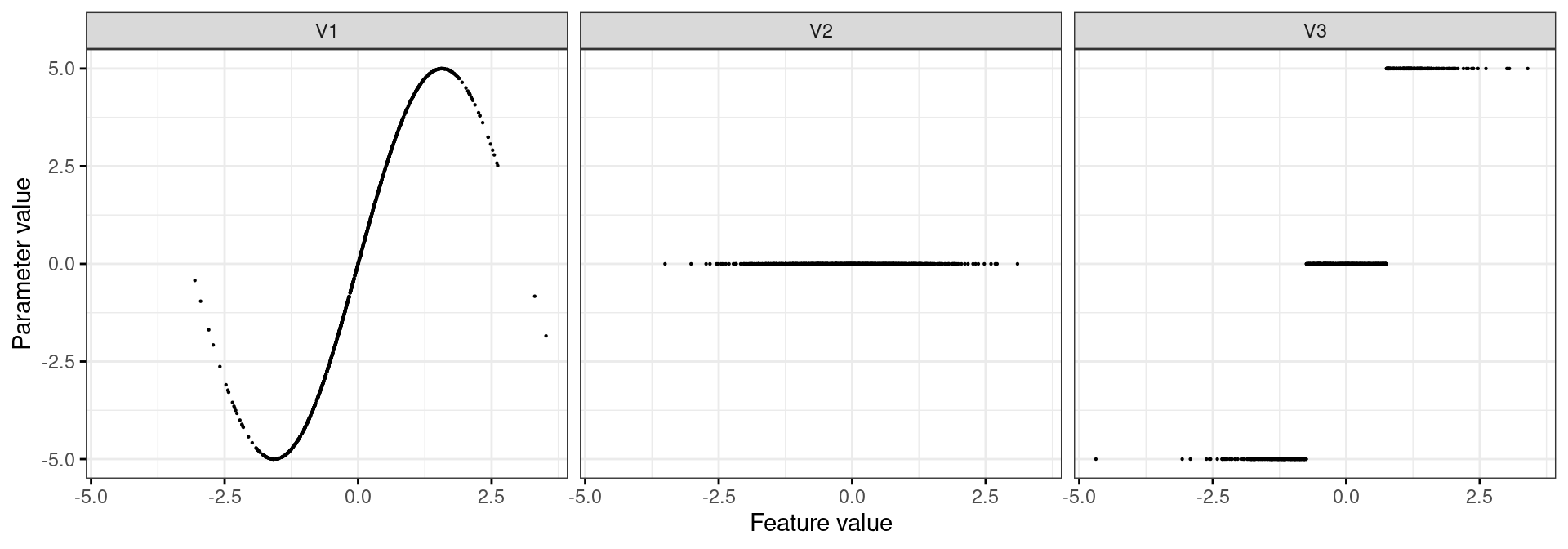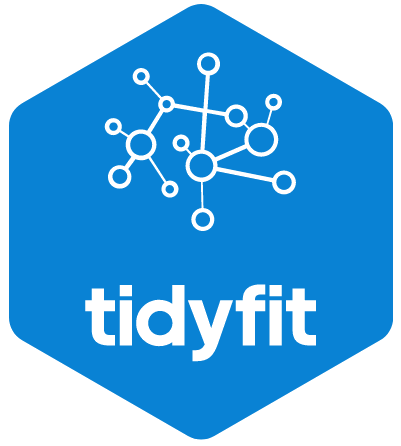## Inference in Neural Networks using an Explainable Parameter Encoder Network

A Parameter Encoder Neural Network (PENN) is an explainable machine learning technique that solves two problems associated with traditional XAI algorithms:

1. It permits the calculation of local parameter distributions. Parameter distributions are often more interesting than feature contributions — particularly in economic and financial applications — since the parameters disentangle the effect from the observation (the contribution can roughly be defined as the demeaned product of effect and observation).
2. It solves a problem of biased contributions that is inherent to many traditional XAI algorithms. Particularly in the setting where neural networks are powerful — in interactive, dependent processes — traditional XAI can be biased, by attributing effect to each feature independently.

At the end of the tutorial, I will have estimated the following highly nonlinear parameter functions for a simulated regression with three variables:A Github version of the code can be found here.

## tidyfit: Extending the tidyverse with AutoMLtidyfit is an R-package that facilitates and automates linear regression and classification modeling in a tidy environment. The package includes several methods, such as Lasso, PLS and ElasticNet regressions, and can be augmented with custom methods. tidyfit builds on the tidymodels suite, but emphasizes automated modeling with a focus on the linear regression and classification coefficients, which are the primary output of tidyfit.

## hfr: An R-Package for Hierarchical Regression Shrinkage

hfr is an R package that implements a novel graph-based regularized regression estimator: the Hierarchical Feature Regression (HFR). The method mobilizes insights from the domains of machine learning and graph theory to estimate robust parameters for a linear regression, constructing a supervised feature graph that decomposes parameters along its edges. The graph adjusts first for common variation and successively incorporates idiosyncratic patterns into the fitting process.

The result is group shrinkage of the parameters, where the extent of shrinkage is governed by a hyperparameter kappa that represents the size of the feature graph. At kappa = 1 the regression is unregularized resulting in OLS parameters. At kappa < 1 the graph is shrunken, reducing the effective model size and regularizing the regression.

## VECM + Neural Network: A semiparametric model of cointegrated data

In this article, I explore a method of nonlinear time series estimation, which combines elements of an artifical neural network (NN) and a vector error correction model (VECM). The aim is to develop a semiparametric VECM, which is capable of modelling nonlinear short-run behaviour of an unknown functional form, while retaining the ability to draw inferential conclusions about the long-run equilibrium behaviour of the data. This approach is particularly useful for data periods including financial crises, multiple regimes and other nonlinear characteristics, which are difficult to handle in a purely linear setting.

The artificial neural network (NN) is a powerful tool for modeling nonlinear empirical relationships. Various authors demonstrate the so-called Universal Approximation Theorem (see for instance Hornik (1991)), proving that single-layer neural networks can approximate any arbitrary function. This makes it an elegant alternative to other nonlinear approaches — particularly, when the functional form of the data-generating process (DGP) is unknown.

Using artificial neural networks to capture nonlinearities in time series data has received a fair amount of attention in the past. Autoregressive neural network (AR-NN) models are well established and have been applied broadly (see Enders (2015)). Various studies compare the performance of multivariate neural network (VAR-NN) models against standard vector autoregression models (see Wutsqa, Subanar, and Sujuti (2006) and Aydin and Cavdar (2015)). Generally, NN-based models exhibit superior performance for prediction purposes, while this comes at the expense of model inference, given the “black-box” nature of the NN component. As always, there is no free lunch in econometrics.